# Second law of thermodynamics

The second law of thermodynamics for a reversible change (that is to say that the system evolves through a succession of equilibrium states) can be written as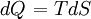$\left.dQ\right.=TdS$

Thus for a closed system, having a fixed number of atoms/molecules, one has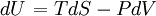$\left.dU\right.=TdS -PdV$

where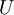$U$ is the internal energy. For an open system: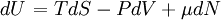$\left.dU\right.=TdS -PdV + \mu dN$

where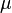$\mu$ is the chemical potential.

For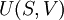$U(S,V)$ one has the following total differential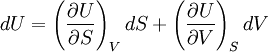$dU=\left(\frac{\partial U}{\partial S}\right)_V dS + \left(\frac{\partial U}{\partial V}\right)_S dV$